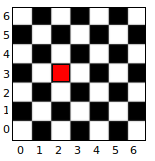Directi  Placement Paper   Candidate Experiences   DCE Delhi-11 Aug 2010

#### Directi  Placement Paper   Candidate Experiences   DCE Delhi-11 Aug 2010

• Posted by  FreshersWorld
7 Jan, 2012

## Know the Contest

 Types of Questions Multiple Choice Multiple Choice, with one or more correct choices (this type is called Multiple Answers). You must have all the right choices marked to get credit for the question Open Ended, where you are required to type the exact answer Time And will remain active for 75 minutes Categories You will have questions in 3 categories: 1min, 3min, 10min. The category name is indicative of the amount of time you are expected to spend on that question Scoring 1min - 1 point 3min - 3 points 10min - 10 points There is no negative marking for incorrect answers

Some of them is here:

1. What is the big-O time complexity of func(p)?
C++ code follows.
int get_power(int a, int b)
{
if(!b) return 1;
if(b%2) return a * get_power(a, b/2);
return get_power(a, b/2);
}
int func(int p)
{
int sum = 0;
for(int i = 1; i <= p; ++i) {
sum += get_power(i, 5);
}
return sum;
}
a. O(p log5)
b. O(plogp)
c. O(logp)
d. O(p)
e. O(p + logp)

2.Given a number N, generate a sequence S such that
S[ 0 ] = N
S[ i+1 ] = (3 * S[ i ] + 1) / 2 if S[ i ] is odd,
= S[ i ] / 2, if S[ i ] is even,
till you reach 1.
For example for N = 5, the sequence is 5, 8, 4, 2, 1
Given two numbers 20 and 35, generate the sequence S for A
and B, and find the number where the two sequences meet.
a.20
b.30
c.35
d.40

If at least two of the statements below
are false, what is the probability that
statement 2 is true?
1. A is true.
2. B is true.
3. A is false and B is true.
a.0
b.0.25
c.0.5
d.0.75
e.1

4.What is the value of p, in main()? C code follows.
char* rev(char s[ ])
{
for(int i = 0, n = strlen(s); s[ i ]; ++i)
{
char c = s[ i ];
s[ i ] = s[ n-1-i ], s[ i ] = c;
}
return s;
}
int main()
{
char s[ ] = "uncommon ideas!";
char *p = rev(s);
}
a. !saedi nommocnu
b. ideas! uncommon
c. uncommon ideas!
d. nommocnu !saedi

5.Given 4 processes with their entering
time and execution time as follows :
Process
Name
Entering
Time
Execution
Time
P1 2 10
P2 3 8
P3 6 4
P4 10 6
A CPU following the shortest job first
algorithm(preemptive) is going to execute
them.
A shortest job first algorithm will execute
a process with the smallest CPU burst
first, because it is preemptive, If a
process is in excution and another
process comes in with CPU burst being
smaller than the remaining execution
time of the currently executing process,
then the new process will be alloted the
CPU and the process currently executing
will be sent to the waiting queue.
For the given processes above Calculate
the average waiting time.
a. 7.25
b. 6.75
c. 5.25
d. 6.25
e. None of the above

6. Given below is the post-order traversal of
a binary search tree. Which of the
following is its pre-order traversal?
(6, 5, 12, 13, 11, 15, 14, 20, 16, 10)
a. 10, 5, 6, 16, 14, 11, 13, 12, 15, 20
b. 10, 5, 6, 15, 14, 13, 11, 12, 16, 20
c. 10, 5, 6, 14, 13, 11, 12, 16, 15, 20
d. 10, 5, 6, 16, 13, 11, 12, 14, 15, 20

7. Insert the numbers in the sequence "8, 5, 1, 4, 2, 10, 12, 7, 3" into a min-heap. The order of insertion is as given. The insertion happens as described here.

If the root is said to be at level 1, the root's children at level 2, and so on, what is the level in which 8 occurs in the heap?

·         a. 1

·         b. 2

·         c. 3

·         d. 4

·         e. 5

8.

You are given a wall that is 50m wide and a rope that is 20m in length. Place the two ends of the rope on the wall and form a figure such that the enclosed area between the wall and the rope is maximized. What is this area?

·         a. 63.63

·         b. 127

·         c. 50

·         d. 127.26

·

9. Two players are playing a game on a checker board (see image below). There is a queen at (i,j) and it can only move such that either "i" or "j" or both decrease (by any amount), that is, it can move only left, or down, or diagonally left-down. The players take turns moving the queen, and the player who first reaches origin wins. Which (i,j) values will result in a win for the first player, among the following? Assume that both players play optimally.
You cannot leave the board.Optimal Play: A player is said to make an "optimal move" if he has considered all his moves, and all the opponent's and his subsequent moves till one of them wins. An optimal player chooses his current move assuming that the opponent will force him into a losing position, if he can.

Example: If the queen is initially at (2,1), your possible moves are to (1,1), (0,1), (2,0) or (1,0). Every one of these moves will make the opponent make his next move to (0,0) and hence (2,1) is a losing position for the first player.

The following image shows a checker board with the cell (2,3) marked in red.

a.
4,5

·         b. 5,3

·         c. 2,3

·         d. 3,4

·         e. 3,5